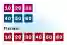# C# Flatten Array (Convert 2D to 1D)

Use a method to flatten 2D arrays into 1D arrays. Benchmark flattened array performance.

Flatten array. A multidimensional array can be flattened. This transformation yields a single-dimensional array—one that is simpler and faster.Notes, 1D arrays. Flattened 2D arrays are ideal for interop with other languages. They can make method signatures simpler and easier to maintain.

An example. We introduce the To1DArray method, which receives a 2D int array and returns a flattened form. The result is a 1D array—we will need to access its elements with an expression.Int Array2D Array
Step 1: We access the 2D array's total element count—the Length property on a 2D returns this value.
Step 2: We copy the 2D array elements into a 1D array. We loop over each dimension.
Step 3: Here we return the 1D array. To access elements with 2 indexes, we will need to multiply the first index.C# program that converts 2D array into 1D array using System; class Program { static int[] To1DArray(int[,] input) { // Step 1: get total size of 2D array, and allocate 1D array. int size = input.Length; int[] result = new int[size]; // Step 2: copy 2D array elements into a 1D array. int write = 0; for (int i = 0; i <= input.GetUpperBound(0); i++) { for (int z = 0; z <= input.GetUpperBound(1); z++) { result[write++] = input[i, z]; } } // Step 3: return the new array. return result; } static void Main() { int[,] elements = { { 10, 20, 30 }, { 40, 50, 60 } }; // Convert 2D array to 1D array. int[] result = To1DArray(elements); // Write flat array. foreach (int value in result) { Console.WriteLine("ELEMENT: {0}", value); } } } Output ELEMENT: 10 ELEMENT: 20 ELEMENT: 30 ELEMENT: 40 ELEMENT: 50 ELEMENT: 60

Index multiplication. Here we consider how to access a flattened array as a 2D array. You multiply the first coordinate by the width, and then add the second coordinate.
Note: To use a jagged array, you must have an array of references to arrays. 2D arrays have significant performance penalties.
Jagged Arrays
Example: This code creates, assigns to and finally displays a 2D array and its equivalent flattened array.
C# program that uses flattened array using System; class Program { static void Main() { while (true) { Console.WriteLine("Enter height: [4+]"); int height = int.Parse(Console.ReadLine()); Console.WriteLine("Enter width: [10+]"); int width = int.Parse(Console.ReadLine()); // // TWO-DIMENSIONAL ARRAY // int[,] twoDimensional = new int[height, width]; // Assign cell 1, 6 twoDimensional[1, 6] = 5; // Assign cell 3, 9 twoDimensional[3, 9] = 9; // Assign cell at 2, 3 twoDimensional[2, 3] = 1; // Display for (int i = 0; i < height; i++) { for (int a = 0; a < width; a++) { Console.Write(twoDimensional[i, a]); } Console.WriteLine(); } Console.WriteLine(); // // FLATTENED ARRAY // int[] oneDimensional = new int[width * height]; // Assign cell 1, 6 oneDimensional[1 * width + 6] = 5; // Assign cell 3, 9 oneDimensional[3 * width + 9] = 9; // Assign cell at 2, 3 oneDimensional[2 * width + 3] = 1; // Display for (int i = 0; i < height; i++) { for (int a = 0; a < width; a++) { Console.Write(oneDimensional[i * width + a]); } Console.WriteLine(); } } } }

Notes, output. The example has some complexity. We see some output from the program that demonstrates how the multiplication results in correct output.
Possible output: Enter height: [4+] 4 Enter width: [10+] 10 0000000000 0000005000 0001000000 0000000009 0000000000 0000005000 0001000000 0000000009 Enter height: [4+] 5 Enter width: [10+] 15 000000000000000 000000500000000 000100000000000 000000000900000 000000000000000 000000000000000 000000500000000 000100000000000 000000000900000 000000000000000 Enter height: [4+] 6 Enter width: [10+] 12 000000000000 000000500000 000100000000 000000000900 000000000000 000000000000 000000000000 000000500000 000100000000 000000000900 000000000000 000000000000

Notes, array access. Suppose we want to change the accesses of a 2D array into flattened, 1D array accesses. We can change them as shown in this table.
Flattened array index computation: array[(Y coordinate * width) + X coordinate] 2D array index computation: array[Y coordinate, X coordinate]

A summary. We can create, assign values to, and display flattened arrays. This is useful for performance, memory reduction, and interoperability with other systems.

Summary, continued. In every application I have applied this technique, performance has improved. Flattened arrays are ideal for hashtable buckets as well as tree structures.Array

Home

© 2007-2020 sam allen. send bug reports to info@dotnetperls.com.